# Exercise E9.3 Areas of Parallelograms and Triangles NCERT Solutions Class 9

Go back to  'Areas of Parallelograms and Triangles'

## Chapter 9 Ex.9.3 Question 1

In the given figure, $$E$$ is any point on median $$AD$$ of a $$\Delta ABC$$. Show that ar ($$ABE=$$) ar ($$ACE$$)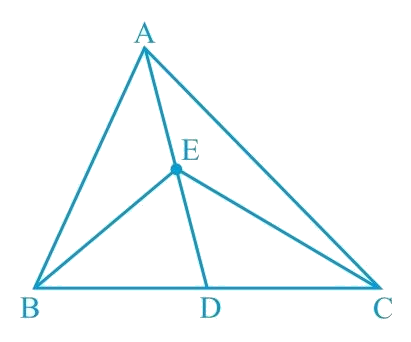### Solution

What is known?

$$E$$ is any point on median $$AD$$ of a $$\Delta ABC$$ .

What is unknown?

How we can show that $$ar (ABE) = ar (ACE)$$

Reasoning:

We know that median divides a triangle in two triangles of equal areas. $$AD$$ is median for triangle $$ABC$$ and $$ED$$ is median of triangle $$EBC$$. Using these facts, we can show the required result.

Steps:

$$AD$$ is the median of $$\Delta ABC$$. Therefore, it will divide $$\Delta ABC$$ into two triangles of equal areas.

Therefore

\begin{align}\text { Area }\!(\Delta ABD)\!=\!\!\text { Area }\!(\Delta ACD)\!\ldots (1) \end{align}

ED is the median of $$\Delta EBC$$

Therefore

\begin{align}\text { Area }\!(\Delta EBD)\!=\!\!\text { Area }\!(\Delta ECD)\!\ldots (2) \end{align}

On subtracting Equation ($$2$$) from Equation ($$1$$), we obtain

\begin{align}\begin{bmatrix}{\text{Area}}\left( {{{\Delta }}{{ABD}}} \right)\\-{\text{Area}}\left( {{{EBD}}} \right)\end{bmatrix} &\!=\!\begin{bmatrix} {\text{ Area }}\left( {{{\Delta }}{{ACD}}} \right)\\ -{\text{Area}}\left( {{{\Delta }}{{ECD}}} \right){{ }}\end{bmatrix}\\{\text{Area }}\left( {{{\Delta }}{{ABE}}} \right)& \!=\! {\text{ Area }}\left( {{{\Delta }}{{ACE}}} \right)\end{align}

## Chapter 9 Ex.9.3 Question 2

In a triangle $$ABC$$, $$E$$ is the mid-point of median$$AD$$. Show that ar \begin{align}(\Delta BED) = \frac {1}{4} \,ar \,(\Delta ABC)\end{align}.

### Solution

What is known?

$$E$$ is the mid-point on median $$AD$$ of a $$\Delta ABC$$ .

What is unknown?

How we can show that $${ar}\left( \!\Delta BED \right)= \frac{1}{4}\,ar\left(\Delta ABC \right)$$ ,

Reasoning:

We know that median divides a triangle in two triangles of equal areas. $$AD$$ is median for triangle $$ABC$$ and $$BE$$ is median of triangle $$ABD$$. Using these facts, we can show the required result.

Steps

Given: $$A$$ $$\Delta ABC$$, $$AD$$ is the median and $$E$$ is the mid-point of median $$AD$$.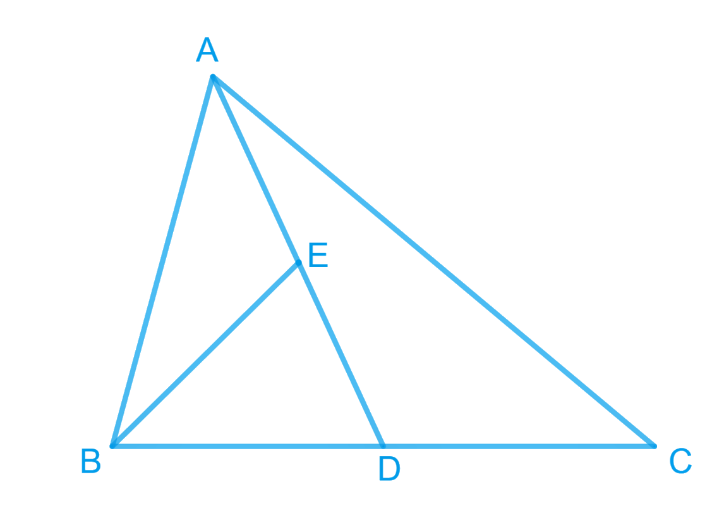To prove: \begin{align} ar (\Delta BED) = \frac {1}{4} ar (\Delta ABC)\end{align}

Proof: In $$\Delta ABC$$, $$AD$$ is the median.

\begin{align}\therefore ar (\Delta ABD) = ar (\Delta ADC)\end{align}

[$$\therefore$$ Median divides a $$\Delta$$  into two $$\Delta$$s of equal area].

\begin{align}ar (\Delta ABD)\!\!=\!\frac {1}{2} ar (ABC) \ldots(1)\end{align}

In $$\Delta ABD$$, $$BE$$ is the median.

\begin{align}\therefore{{{ar }}\left( {{{\Delta BED}}} \right){{ = ar }}\left( {{{\Delta BAE}}} \right)}\end{align}

\begin{align}{\therefore {{ar }}\left( {{{\Delta BED}}} \right){{ = }}\frac{{{1}}}{2}{{ ar}}\left( {{{\Delta ABD}}} \right)}\end{align}

\begin{align}{{ar}}\left( {{{\Delta BED}}} \right)\!\!&=\!\!\frac{{{1}}}{2}\left[ {\frac{{{1}}}{2}{{ar}}\left( {{{\Delta ABC}}} \right)} \right] \dots{\rm{ From (i)}}\end{align}

$\therefore {\rm{ar }}\left( {{{\Delta BED}}} \right){{ = }}\frac{{{1}}}{4}{{ ar }}\left( {{{\Delta ABC}}} \right)$

## Chapter 9 Ex.9.3 Question 3

Show that the diagonals of a parallelogram divide it into four triangles of equal area.

### Solution

What is known?

Parallelogram and its diagonals.

What is unknown?

How we can show that the diagonals of a parallelogram divide it into four triangles of equal area.

Reasoning:

We know that diagonals of a parallelogram bisect each other it means we will get mid- point for both the diagonals through which medians will be formed. Also, median divides the triangle in two triangles of equal areas. By using these observations, we can show the required result.

Steps: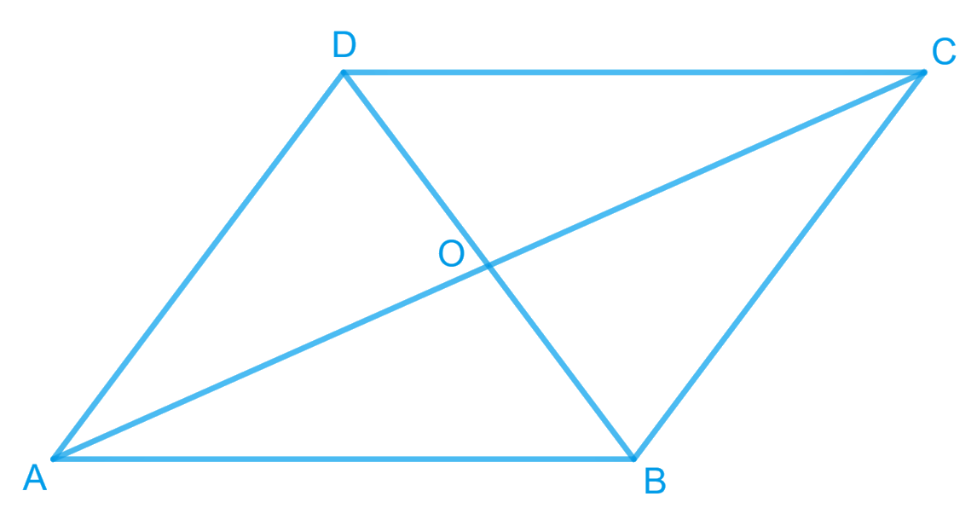We know that the diagonals of a parallelogram bisect each other.

Therefore, $$O$$ is the mid-point of $$AC$$ and $$BD$$.

$$BO$$ is the median in $$\Delta ABC$$. Therefore, it will divide it into two triangles of equal areas.

Therefore

$\text{ ar }\!\!(\Delta AOB)\!\!= \!\!\text{ar } (\Delta BOC) \ldots (1)$

In $$\Delta BCD$$, $$CO$$ is the median.

Therefore

$\text{ ar }\!(\Delta BOC)\!\!=\!\!\text{ar } (\Delta COD) \ldots(2)$

Similarly,

Therefore

$\text{ ar }\!(\Delta COD)\!=\!\text{ar }\!(\Delta AOD) \ldots(3)$

From Equations ($$1$$), ($$2$$), and ($$3$$), we obtain

\begin{align}\text{Area } (\Delta AOB) &= \text{Area } (\Delta BOC)\\& =\text{Area } (\Delta COD)\\& = \text{Area } (\Delta AOD)\end{align}

Therefore, it is evident that the diagonals of a parallelogram divide it into four triangles of equal area.

## Chapter 9 Ex.9.3 Question 4

In the given figure, $$ABC$$ and $$ABD$$ are two triangles on the same base $$AB$$. If line-segment $$CD$$ is bisected by $$AB$$ at $$O$$, show that ar ($$ABC = ar (ABD$$).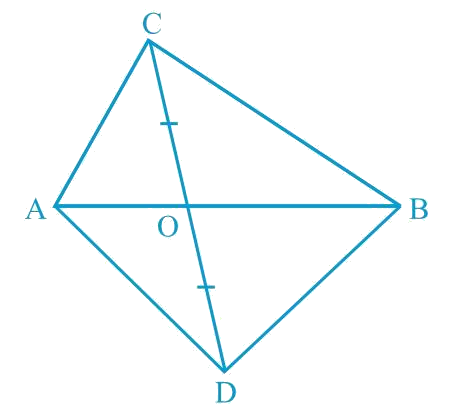### Solution

What is known?

In the given figure, $$ABC$$ and $$ABD$$ are two triangles on the same base $$AB$$. Line-segment $$CD$$ is bisected by $$AB$$ at $$O.$$

What is unknown?

How we can show that $$ar (ABC) = ar (ABD).$$

Reasoning:

$$CD$$ is bisected by $$AB$$ at O means O is the mid-point of $$CD$$. $$AO$$ and $$BO$$ are medians of triangles $$ADC$$ and $$BDC$$. Also, median divides the triangle in two triangles of equal areas. By using these observations, we can show the required result.

Steps:

Consider $$\Delta ACD$$.

Line-segment $$CD$$ is bisected by $$AB$$ at $$O$$. Therefore, $$AO$$ is the median of $$\Delta ACD$$.

$\therefore { ar }\!(\Delta ACO)\!=\!{ar}\!(\Delta ADO) \ldots (1)$

Considering $$\Delta BCD$$, $$BO$$ is the median.

$\therefore { ar }\!(\Delta BCO)\!=\!{ar}\!(\Delta BDO) \ldots(2)$

Adding Equations ($$1$$) and ($$2$$), we obtain

$\begin{array}{l}\left[ \begin{array}{l}{{ar}}(\Delta ACO) + \\{{ar}}(\Delta BCO)\end{array} \right] = \,\left[ \begin{array}{l}{\rm{ar}}(\Delta ADO) + \\{{ar}}(\Delta BDO)\end{array} \right]\\ \Rightarrow {{ar}}\left( {\Delta {{ABC}}} \right)\, = \,{{ar}}\left( {\Delta {{ABD}}} \right)\end{array}$

## Chapter 9 Ex.9.3 Question 5

$$D$$, $$E$$ and $$F$$ are respectively the mid-points of the sides $$BC$$, $$CA$$ and $$AB$$ of a $$\Delta ABC$$. Show that:

(i) $$BDEF$$ is a parallelogram

(ii) \begin{align}{ar } (DEF) = \frac { 1 } { 4 } { ar } (ABC)\end{align}

(iii) \begin{align}{ar } (BDEF) = \frac { 1 } { 2 } { ar } (ABC)\end{align}

### Solution

What is known?

$$D$$, $$E$$ and $$F$$ are respectively the mid-points of the sides $$BC$$, $$CA$$ and $$AB$$ of a $$\Delta \text{ABC}$$

What is unknown?

How we can show that

(i) $$BDEF$$ is a parallelogram.

(ii)$${ar }\left( {DEF} \right){ = }\frac{{1}}{{4}}{ar }\left( {ABC} \right)$$

(iii) $${ar }\left( {BDEF} \right){ = }\frac{{1}}{{2}}{ar }\left( {ABC} \right)$$

Reasoning:

Line joining the mid-points of two sides of a triangle is parallel to the third and half of its length. If one pair of opposite side in quadrilateral is parallel and equal to each other then it is a parallelogram. Diagonals divides the parallelogram in two triangles of equal areas.

Steps:

(i) $$F$$ is the mid-point of $$AB$$ and $$E$$ is the mid-point of $$AC$$.

\begin{align} \therefore \; FE \| BC \text { and } FE = \frac { 1 } { 2 } BC \end{align}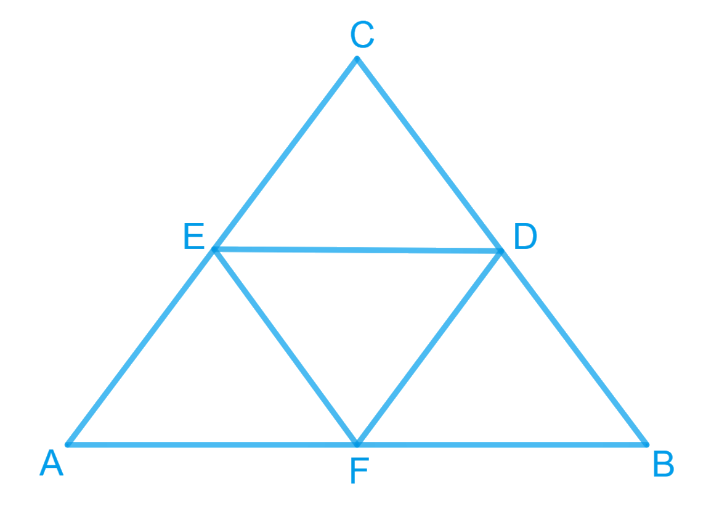Line joining the mid-points of two sides of a triangle is parallel to the third and half of its length.

\begin{align} \therefore FE \| BD \,[BD \text { is the part of } BC]\end{align}

\begin{align} \text { Also, } D \text { is the mid-point of BC. }\end{align}

$\therefore {{BD = }}\frac{{{1}}}{2}{{ BC}}$

\begin{align} FE = BD\end{align}

Now  $$FE\|BD$$ and $$FE = BD$$

Since one pair of opposite side in quadrilateral $$BDEF$$ is parallel and equal to each other

Therefore, $$BDEF$$ is a parallelogram.

(ii) $$BDEF$$ is a parallelogram.

\begin{align} \therefore { ar } (\Delta BDF) = {ar } (\Delta DEF) \ldots (i) \end{align}

[The diagonal $$DF$$ of the parallelogram $$BDEF$$ divides it in two triangles $$BDF$$ and $$DEF$$ of equal area]

Similarly,$$DCEF$$ is also parallelogram.

\begin{align} \therefore { ar } (\Delta DEF)\!=\! {ar } (\Delta DEC)\; \ldots (ii)\end{align}

Also, $$AEDF$$ is a parallelogram.

\begin{align} \therefore\!{ ar } (\Delta AFE)\!=\!{ar } (\Delta DEF) \;\ldots (iii)\end{align}

From equations (i), (ii) and (iii),

\begin{align} (\Delta DEF)& = { ar } (\Delta BDF)\\&= { ar } (\Delta DEC) \\&= { ar } (\Delta AFE)\;\ldots (iv) \end{align}

Now,

\begin{align}&{ar}(\Delta ABC)\\&\!=\!\left[ \begin{array} {ar}(\Delta DEF)\!+\!{ar}(\Delta BDF)+ \\ {ar}(\Delta DEC)\!+\!{ar}(\Delta {AFE})\ \\\end{array} \right]\!\ldots\! ({v})\end{align}

\begin{align}&{{ar}}(\Delta ABC)\\&\!=\!\left[ \begin{array}{l}{{ ar }}(\Delta DEF)\!+\!{{ ar }}(\Delta DEF)\!+\!\\{{ ar }}(\Delta DEF)\!+\!{{ ar }}(\Delta DEF)\end{array} \right]\end{align}

$${\left[ {{{Using }}\left( {{{iv}}} \right){{ \& }}\left( {{v}} \right)} \right]}$$

\begin{align} & {ar } (\Delta ABC) = 4 \times { {ar } (\Delta DEF) } \\ & {ar } (\Delta DEF) = \frac { 1 } { 4 } { ar } (\Delta ABC) \end{align}

(iii)

\begin{align} {ar } (\| gm\; BDEF) &= {ar } (\Delta BDF) + {ar } (\Delta DEF)\\&= {ar } ( \Delta DEF) + {ar } (\Delta DEF) \\\text{ Using } (iv) \end{align}

\begin{align} {ar } (\| { gm } BDEF) &\!=\!2 { ar }\!\!(\Delta DEF) \\ { ar } (\| { gm } BDEF) &\!=\! 2\!\times\!\frac { 1 } { 4 }\!\! { ar }\!\!(\Delta ABC) \\\! { ar }\!( \| { gm } BDEF) &\!=\! \frac { 1 } { 2 } { ar }\!(\Delta ABC) \end{align}

## Chapter 9 Ex.9.3 Question 6

In the given figure, diagonals $$AC$$ and $$BD$$ of quadrilateral $$ABCD$$ intersect at $$O$$ such that $$OB =OD$$. If $$AB = CD$$, then show that:

(i) $$\text { ar } (DOC) = \text {ar } (AOB)$$

(ii) $$\text { ar } (DCB) = \text {ar } (ACB)$$

(iii) $$DA \| CB \text { or } ABCD$$  is a parallelogram.

[Hint: From $$D$$ and $$B$$, draw perpendiculars to $$AC$$.]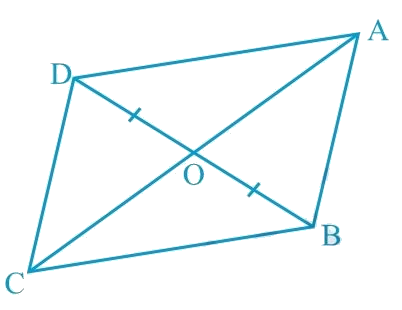### Solution

What is known?

Diagonals $$AC$$ and $$BD$$ of quadrilateral $$ABCD$$ intersect at $$O$$ such that  $$OB =OD.$$Also, $$AB = CD.$$

What is unknown?

How we can show that

(i) $$ar (DOC) = ar (AOB)$$

(ii) $$ar (DCB) = ar (ACB)$$

(iii) $$DA || CB$$ or $$ABCD$$ is a parallelogram.

Reasoning:

We can draw perpendicular from vertices $$B$$ and $$D$$ on diagonal $$AC$$ which will help us to make triangles congruent and we know that congruent triangles are always equal in areas. If two triangles have the same base and equal areas, then these will lie between the same parallels. If in a quadrilateral one pair of opposite sides is equal and the other pair of opposite sides is parallel then it will be a parallelogram.

Steps: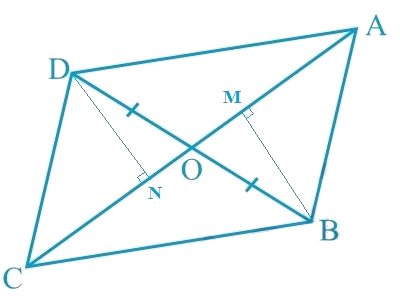Let us draw $$DN \perp AC$$ and $$BM \perp AC$$.

(i) In $$\Delta DON$$ and $$\Delta BOM$$,

\begin{align} & \angle DNO = \angle BMO = 90^{\circ}\end{align} (By construction)

\begin{align} & \angle DON = \angle BOM\; \end{align} (Vertically opposite angles are equal)

\begin{align} & OD = OB\;\end{align} (Given)

By AAS congruence rule,

\begin{align} \Delta DON & \cong \Delta BOM \\ DN &= BM \; \ldots (1)\end{align}

We know that congruent triangles have equal areas.

$\text{ar} (\Delta DON)\!=\!\text{ar} (\Delta BOM) \ldots (2)$

In $$\Delta DNC$$ and $$\Delta BMA$$,

\begin{align} & \angle DNC = \angle BMA = 90^{\circ} \;\end{align} (By construction of perpendicular bisectors)

\begin{align} & CD = AB \;\end{align} (Given)

$$DN = BM$$ [Using Equation (1)]

NOTE: RHS Congruence rule illustrates that, if the hypotenuse and one side of right angled triangle are equal to the corresponding hypotenuse and one side of another right angled triangle; then both the right angled triangle are said to be congruent.

\begin{align} \Delta DNC &\cong \Delta BMA\\text {RHS congru}&\text{ence rule}) \\ \text {ar} (\Delta DNC) &= \text {ar} (\Delta BMA) \ldots(3) \end{align} On adding Equations (\(2) and ($$3$$), we obtain

$\left[ \begin{array}{l}{\rm{ar}}(\Delta DON) + \\{\rm{ar}}(\Delta DNC)\end{array} \right]\!\!=\!\!\left[ \begin{array}{l}{\rm{ar}}(\Delta BOM) + \\{\rm{ar}}(\Delta BMA)\end{array} \right]$

Therefore, $$\text{Area }(\Delta DOC) = \text{Area }(\Delta AOB)$$

(ii) We obtained,

\begin{align}& \text{Area }(\Delta DOC) = \text{Area }(\Delta AOB) \end{align}

$\therefore\left[ \begin{array}{l}{\rm{ar}}(\Delta DOC) + \\{\rm{ar}}(\Delta OCB)\end{array} \right]\!\!=\!\!\left[ \begin{array}{l}{\rm{ar}}(\Delta AOB) + \\{\rm{ar}}(\Delta OCB)\end{array} \right]$

Adding Area $$(\Delta OCB)$$ to both sides

\begin{align} & \therefore \text {ar} (\Delta DCB) = \text {ar} (\Delta ACB) \end{align}

(iii) We obtained,

$\text{ar}(\Delta DCB)\!\!=\!\!\text{ar}(\Delta ACB)$

If Two triangles having the same base (or equal bases) and equal areas lie between the same parallels.

$\therefore DA \|CB \; \ldots (4)$

In quadrilateral $$ABCD$$, one pair of opposite sides is equal $$(AB = CD)$$ and the other pair of opposite sides is parallel $$(DA\|CB)$$.

Therefore, $$ABCD$$ is a parallelogram.

## Chapter 9 Ex.9.3 Question 7

$$D$$ and $$E$$ are points on sides $$AB$$ and $$AC$$ respectively of $$\Delta ABC$$ such that $$\text{ar }(DBC) = \text{ar }(EBC)$$. Prove that $$DE\; \| \;BC$$.

### Solution

What is known?

$$D$$ and $$E$$ are points on sides $$AB$$ and $$AC$$ respectively of such that $$ar (DBC) = ar (EBC).$$

What is unknown?

How we can prove that $$DE || BC.$$

Reasoning:

If two triangles are on common base and have equal areas then they will lie between the same parallel lines.

Steps: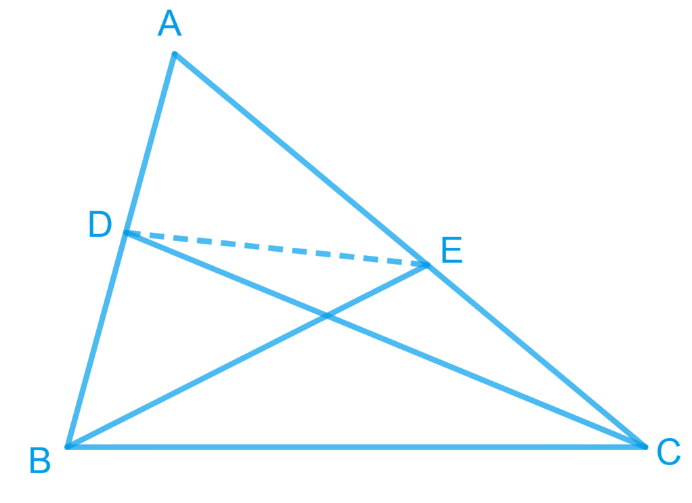Since $$\Delta EBC$$ and $$\Delta DBC$$ are lying on a common base $$BC$$ and also have equal areas, $$\Delta EBC$$ and $$\Delta DBC$$ will lie between the same parallel lines.

$\therefore DE\;\| \;BC$

## Chapter 9 Ex.9.3 Question 8

$$XY$$ is a line parallel to side $$BC$$ of a triangle $$ABC$$. If $$BE\; || \;AC$$ and $$CF\; || \;AB$$ meet $$XY$$ at $$E$$ and $$F$$ respectively, show that $$ar (ABE) = ar (ACF)$$

### Solution

What is known?

$$XY$$ is a line parallel to side $$BC$$ of a triangle $$ABC$$. $$BE || AC$$ and $$CF || AB$$ meet $$XY$$ at $$E$$ and $$F$$ respectively.

What is unknown?

How we can show that $$ar (ABE) = ar (ACF).$$

Reasoning:

if a triangle and parallelogram are on same base and between same parallel lines then area of triangle will be half of area of parallelogram. Also, if two quadrilaterals are on same base and between same pair of parallel lines then both will have equal area. By using these theorems, we can show the required result.

Steps:

Let’s draw points $$M$$ and $$N$$, intersected by line $$XY$$ on sides $$AB$$ and $$AC$$ respectively.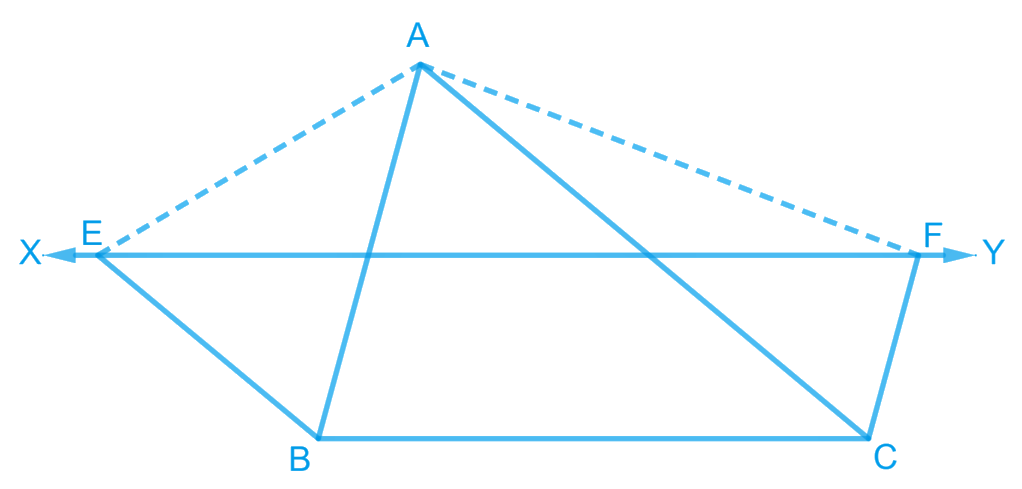It is given that,

$$XY || BC$$ and

$$BE || AC$$

Hence, $$EN || BC$$ and $$BE || CN$$

Therefore, $$BCNE$$ is a parallelogram

It is given that

$$XY || BC$$ and $$FC || AB$$

Hence, $$MF || BC$$ and $$FC || MB$$

Therefore, $$BCFM$$ is a parallelogram.

Parallelograms $$EBCY$$ and $$BCFX$$ are on the same base $$BC$$ and between the same parallels $$BC$$ and $$EF$$.

According to Theorem 9.1 : Parallelograms on the same base and between the same parallels are equal in area.

Therefore

\begin{align}\text {ar}({EBCY})=\text {ar}({BCFX})...\!(1)\end{align}

Consider parallelogram  and $$\Delta AEB$$

These lie on the same base  and are between the same parallels  and .

Therefore\begin{align}\text {ar}(\Delta {ABE})=\frac{1}{2} \text {ar}({EBCY})...\!(2)\end{align}

Also, parallelogram $$\Delta CFXB$$ and $$\Delta ACF$$ are on the same base  and between the same parallels  and .

Therefore

\begin{align}\text {ar}(\Delta {ACF})=\frac{1}{2} \text {ar}({BCFX})...\!(3)\end{align}

From Equations (), (), and (), we obtain

Area ($$\Delta ABE$$)  Area  ($$\Delta ACF$$)

## Chapter 9 Ex.9.3 Question 9

The side $$AB$$ of a parallelogram $$ABCD$$ is produced to any point $$P$$. $$A$$ line through $$A$$ and parallel to $$CP$$ meets $$CB$$ produced at $$Q$$ and then parallelogram $$PBQR$$ is completed (see the following figure). Show that $$ar (ABCD) = ar (PBQR)$$.

[Hint: Join $$AC$$ and $$PQ$$. Now compare area $$(ACQ)$$ and area $$(APQ)$$]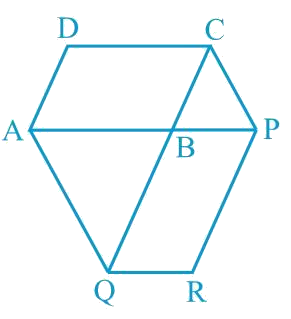### Solution

What is known?

The side AB of a parallelogram $$ABCD$$ is produced to any point $$p$$. A line through A and parallel to $$CP$$ meets $$CB$$ produced at $$Q$$ and then parallelogram $$PBQR$$ is completed.

What is unknown?

How we can show that $$ar (ABCD) = ar (PBQR).$$

Reasoning:

First of all, we can join $$AC$$ and $$PQ$$. Now we can use theorem for triangles $$ACQ$$ and $$AQP$$ if two triangles are on same base and between same pair of parallel lines then both will have equal area. Now we can subtract common area of triangle $$ABQ$$ from both sides. Now we can compare the result with half of which we have to show.

Steps: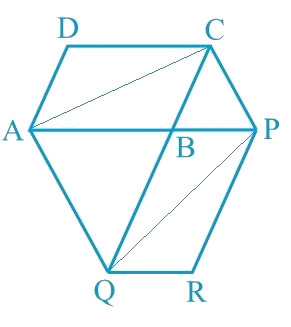Let us join $$AC$$ and $$PQ$$.

$$\Delta ACQ$$ and $$\Delta AQP$$ are on the same base $$AQ$$ and between the same parallels $$AQ$$ and $$CP$$.

According to Theorem 9.2: Two triangles on the same base (or equal bases) and between the same parallels are equal in area.

\begin{align} \text {ar}(\Delta {ACQ})&=\text {ar}(\Delta {APQ})\end{align}

$\,\left[ \begin{array}{l}{\rm{ar}}(\Delta {{ACQ}})\, -\\{\rm{ar}}(\Delta {{ABQ}})\end{array} \right]\!\!=\!\!\left[ \begin{array}{l}{\rm{ar}}(\Delta {{APQ}})\, - \\{{ar}}(\Delta {{ABQ}})\end{array} \right]$

Subtracting Area $$(\Delta {ABQ})$$ on both the sides.

\begin{align} (\Delta {ABC})&=\text {ar}(\Delta {QBP}) ...(1)\end{align}

Since  and  are diagonals of parallelograms  and  respectively,

\begin{align}\text {ar}(\Delta {ABC})&=\frac{1}{2} \text {ar}({ABCD})...(2) \\ \text {ar}(\Delta {QBP})&=\frac{1}{2} {ar(PBQR) } ...(3)\end{align}

From Equations (), (), and (), we obtain

\begin{align}\frac{1}{2} \text {ar}({ABCD})&=\frac{1}{2} \text {ar}(PBQR) \\ \text {ar}({ABCD})&=\text {ar}({PBQR})\end{align}

## Chapter 9 Ex.9.3 Question 10

Diagonals $$AC$$ and $$BD$$ of a trapezium $$ABCD$$ with $$AB\; || \;DC$$ intersect each other at $$O$$. Prove that $$ar (AOD) = ar (BOC)$$.

### Solution

What is known?

Diagonals $$AC$$ and $$BD$$ of a trapezium $$ABCD$$ with $$AB || DC$$ intersect each other at $$O$$.

What is unknown?

How we can prove that $$ar (AOD) = ar (BOC).$$

Reasoning:

We can use theorem for triangles $$DAC$$ and $$DBC$$, if two triangles are on same base and between same pair of parallel lines then both will have equal area. Now we can subtract common area of triangle $$DOC$$ from both sides to get the required result.

Steps: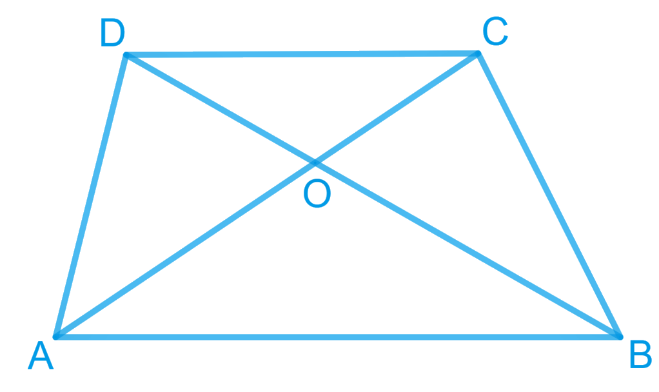It can be observed that \begin{align} (\Delta DAC)\, \rm{and} \,(\Delta DBC)\end{align} lie on the same base $$DC$$ and between the same parallels $$AB$$ and $$CD$$.

According to Theorem 9.2: Two triangles on the same base (or equal bases) and between the same parallels are equal in area.

\begin{align} {ar}\left( \Delta { DAC} \right)&={ar}\left( \Delta { DBC} \right) \\\left[ \begin{array} {ar}\left( \Delta { DAC} \right)- \\ {ar }\left( \Delta { DOC} \right) \\\end{array} \right]&\!\!=\!\!\left[ \begin{array} {ar }\left( \Delta { DBC} \right)- \\ {ar }\left( \Delta { DOC} \right) \\\end{array} \right] \\\end{align}

Subtracting area $$(\Delta{ DOC})$$ from both sides

\begin{align} {ar }\left( \Delta{ AOD} \right)&={ ar }\left( \Delta{ BOC} \right) \\\end{align}

## Chapter 9 Ex.9.3 Question 11

In the given figure, $$ABCDE$$ is a pentagon. $$A$$ line through $$B$$ parallel to $$AC$$ meets $$DC$$ produced at $$F$$. Show that

(i) $$ar (ACB) = ar (ACF)$$

(ii) $$ar (AEDF) = ar (ABCDE)$$

### Solution

What is known?

$$ABCDE$$ is a pentagon. $$A$$ line through $$B$$ parallel to $$AC$$ meets $$DC$$ produced at $$F.$$

What is unknown?

How we can show that

(i) $$ar (ACB) = ar (ACF)$$

(ii) $$ar (AEDF) = ar (ABCDE)$$

Reasoning:

We can use theorem for triangles $$ACB$$ and $$ACF$$, if two triangles are on same base and between same pair of parallel lines then both will have equal area. Now we can add area of quadrilateral $$ACDE$$ on both sides to get the second part required result.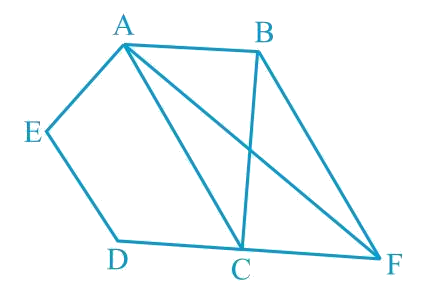Steps:

(i) \begin{align}\rm \Delta ACB\, \rm and \,\Delta ACF\end{align} lie on the same base $$AC$$ and The same parallels $$AC$$ and $$BF$$.

According to Theorem 9.2: Two triangles on the same base (or equal bases) and between the same parallels are equal in area.

\begin{align}\text{Area}\; ( \Delta ACB ) = \text{Area}\; ( \Delta ACF )\end{align}

(ii) It can be observed that

\begin{align} {\text {ar}(\Delta {ACB})}\!=\!{\text {ar}(\Delta {ACF})} \end{align}

$\left[ \begin{array}{l}{{ar}}(\Delta {{ACB}}) + \\{{ar}}({{ACDE}})\end{array} \right] \!\!=\!\!\left[ \begin{array}{l}{{ar}}(\Delta {{ACF}}) + \\{{ar(ACDE) }}\end{array} \right]$

Adding Equal Areas  on both the sides.

\begin{align}\text{ar}\, (ABCDE)\!=\!\text{ar} \,(AEDF)\end{align}

## Chapter 9 Ex.9.3 Question 12

A villager Itwaari has a plot of land of the shape of a quadrilateral. The Gram Panchayat of the village decided to take over some portion of his plot from one of the corners to construct a Health Centre. Itwaari agrees to the above proposal with the condition that he should be given equal amount of land in lieu of his land adjoining his plot so as to form a triangular plot. Explain how this proposal will be implemented.

### Solution

What is known?

Itwaari has a plot of land of the shape of a quadrilateral and some portion of his plot Gram Panchayat use to construct Health Centre. He should be given equal amount of land in lieu of his land adjoining his plot so as to form a triangular plot.

What is unknown?

How this proposal will be implemented.

Reasoning:

Join diagonal $$BD$$ and draw a line parallel to $$BD$$ through point A.Let it meet the extended side $$CD$$ of $$ABCD$$ at point $$E$$.Join $$BE$$ and $$AD$$. Let them intersect each other at O. We can use theorem for triangles $$DEB$$ and $$DAB$$, if two triangles are on same base and between same pair of parallel lines then both will have equal area. Now we can subtract area of triangle DOB from both sides to get the required result.

Steps: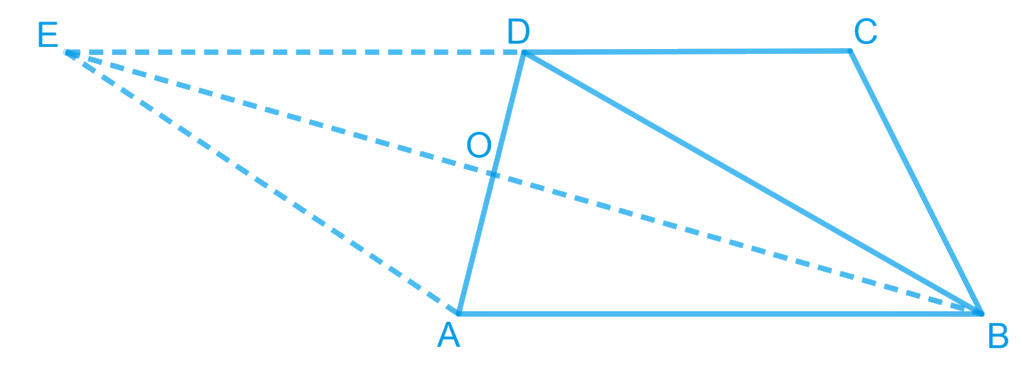Let quadrilateral $$ABCD$$ be the original shape of the field.

The proposal may be implemented as follows.

Join diagonal $$BD$$ and draw a line parallel to $$BD$$ through point $$A$$.

Let it meet the extended side $$CD$$ of $$ABCD$$ at point $$E$$.

Join $$BE$$ and $$AD$$. Let them intersect each other at $$O$$.

Then, portion $$\Delta AOB$$ can be cut from the original field so that the new shape of the field will be . (See figure).

We have to prove that the area of $$\Delta AOB$$ (portion that was cut so as to construct Health Centre) is equal to the area of $$\Delta DEO$$ (portion added to the field so as to make the area of the new field so formed equal to the area of the original field).

It can be observed that $$ΔDEB$$ and $$ΔDAB$$ lie on the same base $$BD$$ and are between the same parallels $$BD$$ and $$AE$$.

According to Theorem 9.2 : Two triangles on the same base (or equal bases) and between the same parallels are equal in area.

\begin{align}{\text{ Area }}(\Delta {{DEB}})&\!\!= \!\!{\text{ Area }}(\Delta {{DAB}})\\\left[ \begin{array}{l}{\text{Area }}(\Delta {{DEB}}) - \\{\text{ Area }}(\Delta {{DOB}})\end{array} \!\right] &\!\!=\!\!\left[ \begin{array}{l}{\text{Area }}(\Delta {{DAB}}) - \\ {\text{Area }}(\Delta {{DOB}})\end{array}\!\right]\\{\text{Area}}\left( {\Delta {{DEO}}} \right)&\!\!=\!\! {\text{Area}}\left( {\Delta {{AOB}}} \right)\end{align}

## Chapter 9 Ex.9.3 Question 13

$$ABCD$$ is a trapezium with $$AB\; || \;DC$$. A line parallel to $$AC$$ intersects $$AB$$ at $$X$$ and $$BC$$ at $$Y$$.

Prove that $$ar (ADX) = ar (ACY)$$.

[Hint: Join $$CX$$.]

### Solution

What is known?

$$ABCD$$ is a trapezium with $$AB || DC$$. A line parallel to $$AC$$ intersects $$AB$$ at $$X$$ and $$BC$$ at $$Y.$$

What is unknown?

How we can prove that $$ar (ADX) = ar (ACY).$$

Reasoning:

We can use theorem for triangles $$ADX$$ and $$ACX$$, if two triangles are on same base and between same pair of parallel lines then both will have equal area. Similarly for triangles we can use for triangles $$ACX$$ and $$ACY$$. Now by comparing both the results, we will get the required result.

Steps: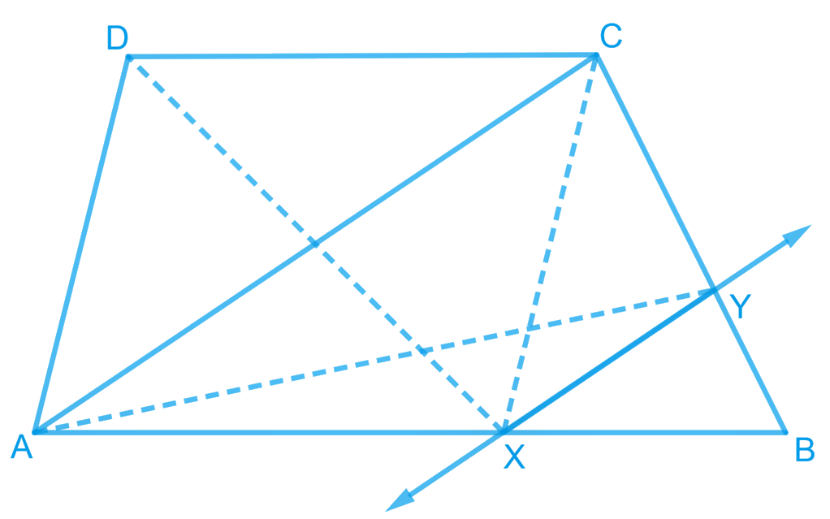It can be observed that \begin{align}\Delta ADX \, \rm {and} \,\Delta ACX \end{align} lie on the same base $$AX$$ and are between the same parallels $$AB$$ and $$DC$$.

According to Theorem 9.2 : Two triangles on the same base (or equal bases) and between the same parallels are equal in area.

\begin{align}\therefore \text {ar }(\Delta {ADX})\!=\!\text {ar}(\Delta {ACX})\dots{(1)}\end{align}

$$\Delta ACY$$ and $$\Delta ACX$$ lie on the same base $$AC$$ and are between the same parallels $$AC$$ and $$XY$$.

According to Theorem 9.2: Two triangles on the same base (or equal bases) and between the same parallels are equal in area.

\begin{align}(\Delta {ACY})\!=\!\text {ar}({ACX})\,\ldots \text (2)\end{align}

From Equations ($$1$$) and ($$2$$), we obtain

\begin{align}{\rm{ar}}\left( {{{\Delta ADX}}} \right)\!=\!{\rm{ar}}\left( {{{\Delta ACY}}} \right)\end{align}

## Chapter 9 Ex.9.3 Question 14

In the given figure, $$AP \;|| \;BQ \;|| \;CR$$. Prove that $$ar (AQC) = ar (PBR)$$.

### Solution

What is known?

$$AP || BQ || CR.$$

What is unknown?

How we can prove that $$ar (AQC) = ar (PBR).$$

Reasoning:

We can use theorem for triangles $$ABQ$$ and $$PBQ$$, if two triangles are on same base and between same pair of parallel lines then both will have equal area. Similarly for triangles we can use for triangles $$BCQ$$ and $$BRQ$$. Now by adding both the results, we will get the required result.

Steps: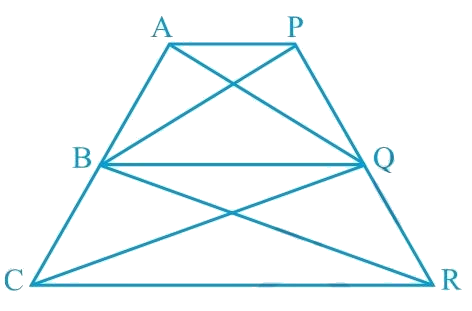Since \begin{align}\rm \Delta ABQ\, \rm and \,\Delta PBQ\end{align} lie on the same base $$BQ$$ and are between the same parallels $$AP$$ and $$BQ$$,

According to Theorem 9.2 : Two triangles on the same base (or equal bases) and between the same parallels are equal in area.

\begin{align}\therefore \text {ar}(\Delta {ABQ})\!=\!\text {ar}(\triangle {PBQ})\dots\text (1)\end{align}

\begin{align} (\Delta BCQ)\, and \,(\Delta BRQ)\end{align} lie on the same base  and are between the same parallels  and .

\begin{align}\therefore \text {ar}(\Delta B C Q)\!=\!\text {ar}(\Delta B R Q) \ldots \text (2)\end{align}

On adding Equations ($$1$$) and ($$2$$), we obtain

$\left[ \begin{array}{l}{{ar}}(\Delta {{ABQ}}) + \\{{ar}}(\Delta {{BCQ}})\end{array} \right] = \left[ \begin{array}{l}{{ar}}(\Delta {{PBQ}})\!+\!\\{{ar}}(\Delta {{BRQ}})\end{array} \right]$

\begin{align} {\therefore \text {ar}(\Delta {AQC})}&\!=\!{ {ar}(\Delta {PBR})}\end{align}

## Chapter 9 Ex.9.3 Question 15

Diagonals $$AC$$ and $$BD$$ of a quadrilateral $$ABCD$$ intersect at $$O$$ in such a way that $$ar (AOD) = ar (BOC)$$. Prove that $$ABCD$$ is a trapezium.

### Solution

What is known?

Diagonals $$AC$$ and $$BD$$ of a quadrilateral $$ABCD$$ intersect at $$O$$ in such a way that $$ar (AOD) = ar (BOC).$$

What is unknown?

How we can prove that $$ABCD$$ is a trapezium.

Reasoning:

We can add area of triangle $$AOB$$ on both sides in the given equal area’s triangle. Now we will get two new triangles of equal areas with common base. Now we can use theorem that if Two triangles having the same base (or equal bases) and equal areas lie between the same parallels.

Steps: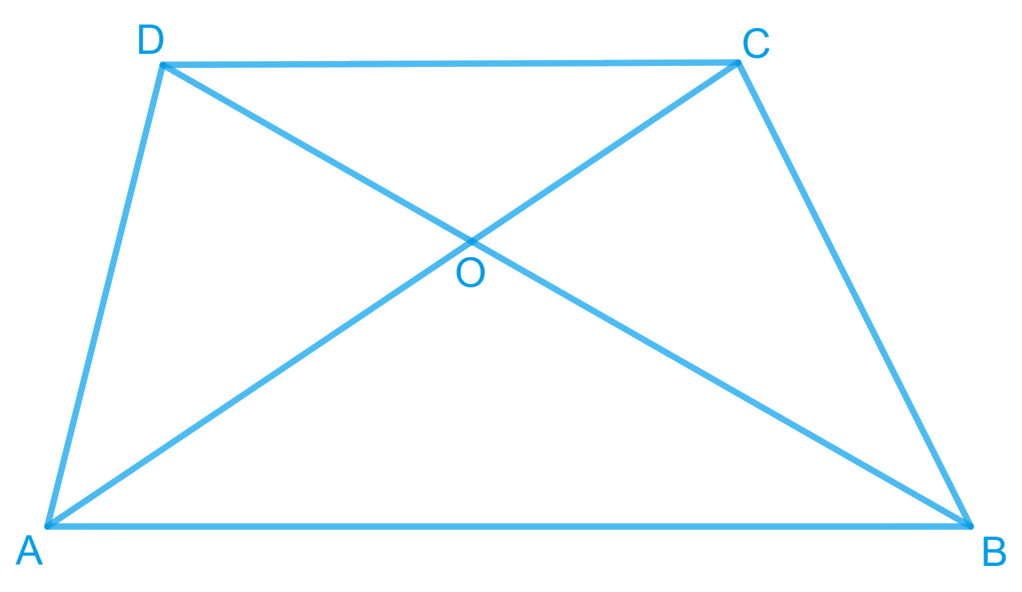It is given that,

\begin{align} {\text { Area }(\Delta AOD)}&={\text { Area }(\Delta BOC)} \end{align}

Area $$(\Delta \,AOD)+$$  Area $$(\Delta \,AOB)=$$ Area $$(\Delta \, BOC)+$$ Area $$(\Delta \,AOD)$$

Adding Area $$(\Delta \, AOB )$$  on both the sides

\begin{align} {\therefore \text { Area }(\Delta ADB)}&={\text { Area }(\Delta ACB)}\end{align}

We know that triangles on the same base having areas equal to each other lie between the same parallels.

Therefore, these triangles, \begin{align} (\Delta ADB)\, {\rm{and}} \,(\Delta ACB)\end{align}, are lying between the same parallels. i.e., $$AB \;|| \;CD$$

According to Theorem $$9.3 :$$ Two triangles having the same base (or equal bases) and equal areas lie between the same parallels.

Therefore, $$ABCD$$ is a trapezium.

## Chapter 9 Ex.9.3 Question 16

In the given figure,

$$ar (DRC)$$ $$= ar (DPC)$$ and $$ar (BDP) = ar (ARC)$$.

Show that both the quadrilaterals $$ABCD$$ and $$DCPR$$ are trapeziums.

### Solution

What is known?

$$ar (DRC) = ar (DPC)$$ and $$ar (BDP) = ar (ARC).$$

What is unknown?

How we can show that quadrilaterals $$ABCD$$ and $$DCPR$$ are trapeziums.

Reasoning:

We know that if Two triangles having the same base (or equal bases) and equal areas lie between the same parallels.

Steps:

It is given that

$$\text{Area} (\Delta DRC )= \text{Area} (\Delta DPC )$$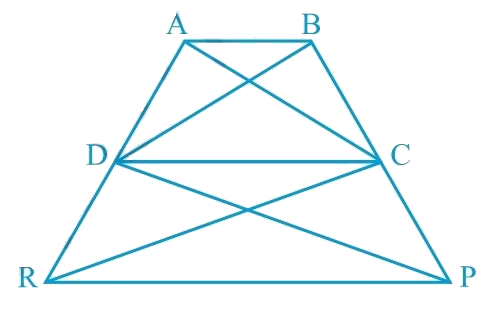As $$\Delta DRC$$ and $$\Delta DPC$$ lie on the same base $$DC$$ and have equal areas, therefore, they must lie between the same parallel lines.

According to Theorem $$9.3$$ : Two triangles having the same base (or equal bases) and equal areas lie between the same parallels.

$\therefore {{DC || RP}}$

Therefore, $$DCPR$$ is a trapezium.

It is also given that

\begin{align}\text { Area }(\Delta {BDP})=\text { Area }(\Delta {ARC})\end{align}

Area $$(\Delta \, BDP)$$ - Area $$(\Delta\, DPC)$$ = Area $$(\triangle \,ARC)$$ -Area $$(\Delta \, DRC)$$

\begin{align} {\therefore \text { Area }(\Delta {BDC})}&={\text { Area }(\Delta {ADC})}\end{align}

Since \begin{align} (\Delta BDC )\, and \,(\Delta ADC)\end{align} are on the same base $$CD$$ and have equal areas, they must lie between the same parallel lines.

According to Theorem $$9.3$$ : Two triangles having the same base (or equal bases) and equal areas lie between the same parallels.

\begin{align}\therefore \quad A B \| C D\end{align}

Therefore, $$ABCD$$ is a trapezium.

Related Sections
Related Sections
Instant doubt clearing with Cuemath Advanced Math Program